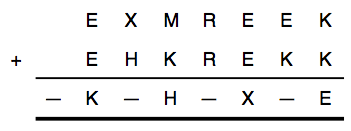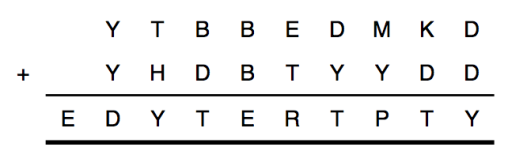# Smudged secret messages

This Riddler is a twist on a classic problem: decoding equations! Here is the paraphrased problem:

The goal is to decode two equations. In each of them, every different letter stands for a different digit. But there is a minor problem in both equations. In the first equation, letters accidentally were smudged and are now unreadable. (These are represented with dashes below.) But we know that all 10 digits, 0 through 9, appear in the equation.What digits belong to what letters, and what are the dashes? In the second equation, one of the letters in the equation is wrong. But we don’t know which one. Which is it?Here is my detailed solution:
[Show Solution]

If you’re just interested in the answers, here they are:
[Show Solution]